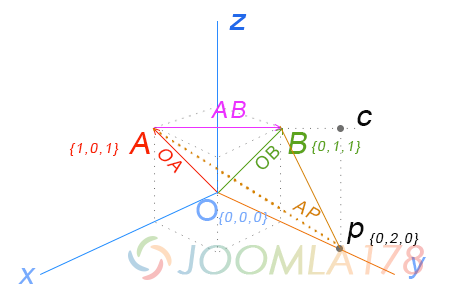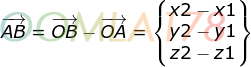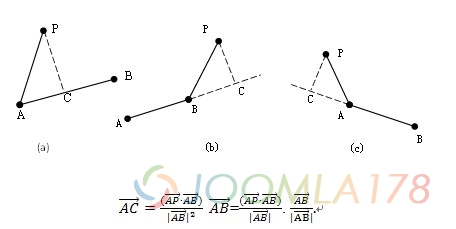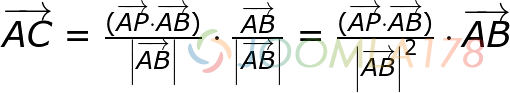# 空间向量的基本计算及Mathjs的实现

### 一、概念理解### 二、计算逻辑和实现

A. 点到点距离``var A = {x:1,y:0,z:1}, B = {x:0, y:1, z:1}var ABDistance = Math.sqrt(Math.pow((B.x - A.x),2) + Math.pow((B.y - A.y),2) + Math.pow((B.z - A.z),2))console.log(ABDistance)1.4142135623730951``

B. 点到线距离• 1、向量AC = 向量AC的模 * 向量AC的单位向量
• 2、因为C点在向量AB上，所以向量AC的单位向量 = 向量AB的单位向量
• 3、向量AC的模 = 向量AP和向量AB的点积 / 向量AB模  （避免使用AP和AB的夹角计算AC的模）• 1、r ≤ 0，则是c图情况，C点则在A的负方向，那么最短距离就是向量AP的模（长度）
• 2、r > 1，则是b图情况，C点则在B的正方向，最短距离就是向量BP的模（长度）
• 3、0 < r < 1，则是a图情况，C点则介于A和B中间，最短距离就是向量CP的模（长度）

``````var A = {x:1,y:0,z:1}
var B = {x:0,y:1,z:1}
var p = {x:0,y:2,z:0}// 定义向量AP和向量ABvar APV = {x: p.x - A.x, y: p.y - A.y, z: p.z - A.z}var ABV = {x: B.x - A.x, y: B.y - A.y, z: B.z - A.z}// 求向量AP和向量AB的内积var cross = APV.x * ABV.x + APV.y * ABV.y + APV.z * ABV.z // 示例结果是3// 求向量AB模的平方，注意求模是平方根var ABPow = Math.pow((B.x - A.x),2) + Math.pow((B.y - A.y),2) + Math.pow((B.z - A.z),2) // 示例结果是2// 根据r的公式计算如下var r = cross / ABPow // 示例结果是 3/2 = 1.5``````

``var PBDistance = Math.sqrt(Math.pow((p.x - B.x),2) + Math.pow((p.y - B.y),2) + Math.pow((p.z - B.z),2)) // 示例结果是1.4142135623730951``

``````var A = {x:1,y:0,z:1}
var B = {x:0,y:1,z:1}
var p = {x:0,y:0.5,z:0}
// 定义向量AP和向量AB
var APV = {x: p.x - A.x, y: p.y - A.y, z: p.z - A.z}
var ABV = {x: B.x - A.x, y: B.y - A.y, z: B.z - A.z}
// 求向量AP和向量AB的内积
var cross = APV.x * ABV.x + APV.y * ABV.y + APV.z * ABV.z // 示例结果是1.5
// 求向量AB模的平方，注意求模是平方根
var ABPow = Math.pow((B.x - A.x),2) + Math.pow((B.y - A.y),2) + Math.pow((B.z - A.z),2) // 示例结果是2
// 根据r的公式计算如下
var r = cross / ABPow // 示例结果是 1.5/2 = 0.75// 得到向量AC
var ACV = {x: (B.x - A.x) * r, y: (B.y - A.y) * r, z: (B.z - A.z) * r} // 结果是{x: -0.75, y: 0.75, z: 0}var C = {x: ACV.x + A.x, y: ACV.y + A.y, z: ACV.z + A.z} // 结果是{x: 0.25, y: 0.75, z: 1}var ACDistance = Math.sqrt(Math.pow((C.x - A.x),2) + Math.pow((C.y - A.y),2) + Math.pow((C.z - A.z),2)) // 结果是1.0606601717798212// 得到p到C的距离var pCDistance = Math.sqrt(Math.pow((p.x - C.x),2) + Math.pow((p.y - C.y),2) + Math.pow((p.z - C.z),2)) // 结果是1.0606601717798212``````

### 三、Mathjs distance方法实现

``math.distance([x, y, z], [x0, y0, z0, a, b, c])math.distance({pointX: 2, pointY: 5, pointZ: 9}, {x0: 4, y0: 6, z0: 3, a: 4, b: 2, c: 0})``

p点为  `{x:0, y:2, z:1}` 验证，代码如下

``var A = {x:1,y:0,z:1}var B = {x:0,y:1,z:1}var p = {x:0,y:2,z:0}math.distance([p.x, p.y, p.z], [A.x, A.y, A.z, B.x - A.x, B.y - A.y, B.z - A.z])1.224744871391589``

``var A = {x:1,y:0,z:1}var B = {x:0,y:1,z:1}var p = {x:0,y:0.5,z:0}math.distance([p.x, p.y, p.z], [A.x, A.y, A.z, B.x - A.x, B.y - A.y, B.z - A.z])1.0606601717798212``

### 四、Mathjs的精简方法

Mathjs同时支持npm包方式导入至工程化项目中。

npm install --save mathjs

``````// 加载mathjs核心库
const core = require('mathjs/core')
// 创建math对象
// 它只包含`import`和`config`方法
const math = core.create()// 导入distance模块math.import(require('mathjs/lib/function/geometry/distance'))// 导入所需模块math.import(require('mathjs/lib/function/arithmetic/add'))````math.import(require('mathjs/lib/function/arithmetic/subtract'))math.import(require('mathjs/lib/function/arithmetic/multiply'))``math.import(require('mathjs/lib/function/arithmetic/divide'))math.import(require('mathjs/lib/function/string/format'))````

MORE>>

MORE>>

MORE>>